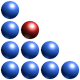# The not-that-useful Definitions of Complexity

“Every few months seems to produce another paper proposing yet another measure of complexity, generally a quantity which can’t be computed for anything you’d actually care to know about, if at all. These quantities are almost never related to any other variable, so they form no part of any theory telling us when or how things get complex, and are usually just quantification for quantification’s own sweet sake”. Read more in: http://cscs.umich.edu/~crshalizi/notebooks/complexity-measures.html. The above mentioned abundance of candidate complexity measures – a clear reflection of the rampant fragmentation in the field – is summarized in: http://en.wikipedia.org/wiki/Complexity as follows: In several scientific fields, “complexity” has a specific meaning:

In computational complexity theory, the time complexity of a problem is the number of steps that it takes to solve an instance of the problem as a function of the size of the input (usually measured in bits), using the most efficient algorithm. This allows to classify problems by complexity class (such as P, NP) such analysis also exists for space, that is, the memory used by the algorithm.

In algorithmic information theory, the Kolmogorov complexity (also called descriptive complexity or algorithmic entropy) of a string is the length of the shortest binary program which outputs that string.

In information processing, complexity is a measure of the total number of properties transmitted by an object and detected by an observer. Such a collection of properties is often referred to as a state.

In physical systems, complexity is a measure of the probability of the state vector of the system. This is often confused with entropy, but is a distinct Mathematical analysis of the probability of the state of the system, where two distinct states are never conflated and considered equal as in statistical mechanics.

In mathematics, Krohn-Rhodes complexity is an important topic in the study of finite semigroups and automata.

In the sense of how complicated a problem is from the perspective of the person trying to solve it, limits of complexity are measured using a term from cognitive psychology, namely the hair limit.
Specified complexity is a term used in intelligent design theory, first coined by William Dembski.

Irreducible complexity is a term used in arguments against the generally accepted theory of biological evolution, being a concept popularized by the biochemist Michael Behe.

Unruly complexity denotes situations that do not have clearly defined boundaries, coherent internal dynamics, or simply mediated relations with their external context, as coined by Peter Taylor.

SDL complexity – the “simple” measure of complexity of Shiner, Davison and Landsberg (SDL). As an SDL classical complexity measure, the quantum complexity measure is defined by the weighted product of the quantum disorder by the quantum order.

And now, ask yourself this: can I use any of these measures to study the evolution of a corporation, of air-traffic, of a market? Can any of these ‘measures’ help identify a complex system and distinguish it from a “simple system”?

www.ontonix.com